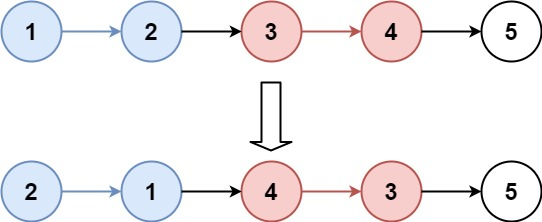# K 个一组翻转链表

## 25. K 个一组翻转链表 (Hard)

• 你可以设计一个只使用常数额外空间的算法来解决此问题吗？
• 你不能只是单纯的改变节点内部的值，而是需要实际进行节点交换。```输入：head = [1,2,3,4,5], k = 2

``````输入：head = [1,2,3,4,5], k = 3

```

```输入：head = [1,2,3,4,5], k = 1

```

```输入：head = , k = 1

```

• 列表中节点的数量在范围 `sz`
• `1 <= sz <= 5000`
• `0 <= Node.val <= 1000`
• `1 <= k <= sz`

[递归] [链表]

### 相似题目

1. 两两交换链表中的节点 (Medium)